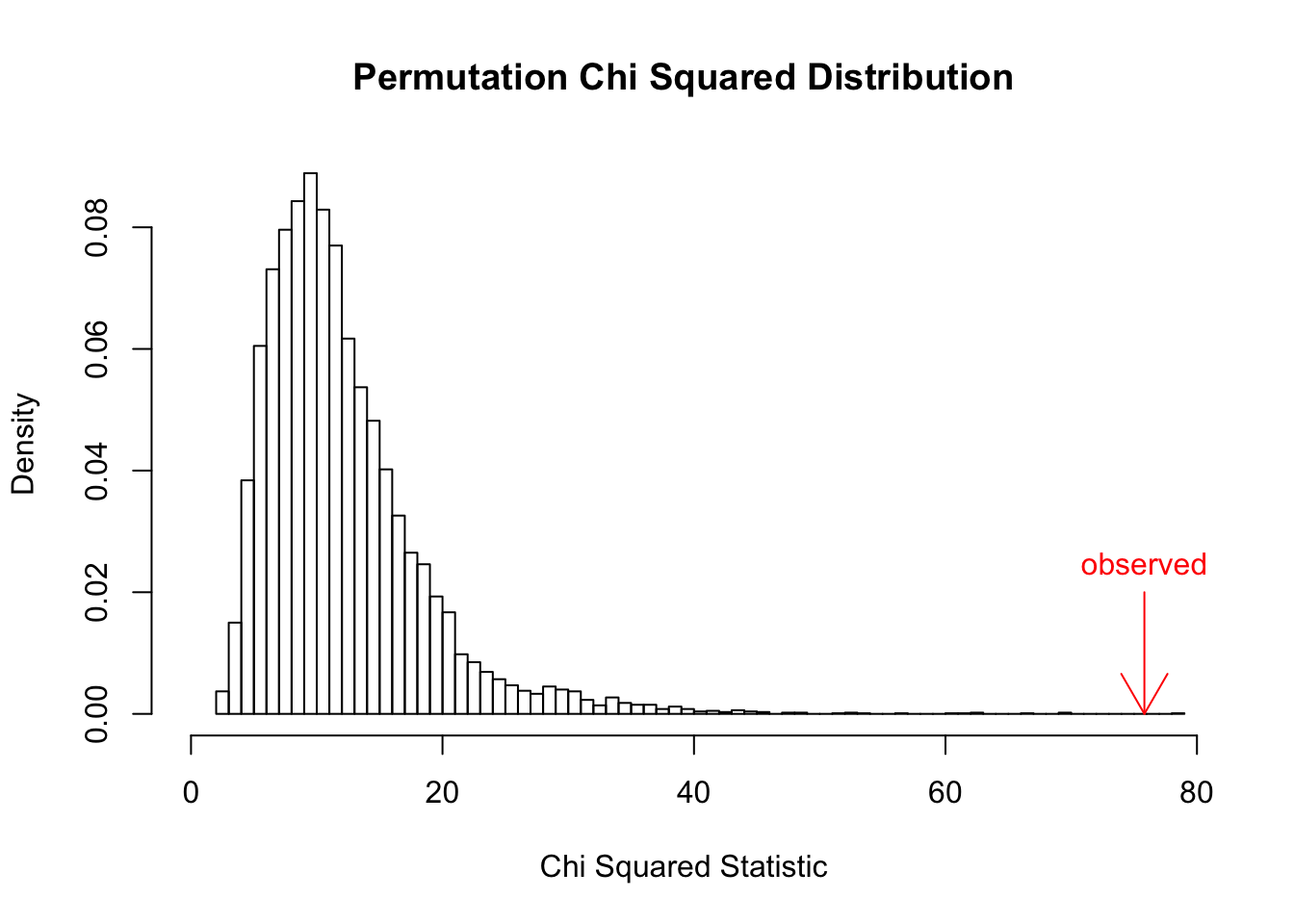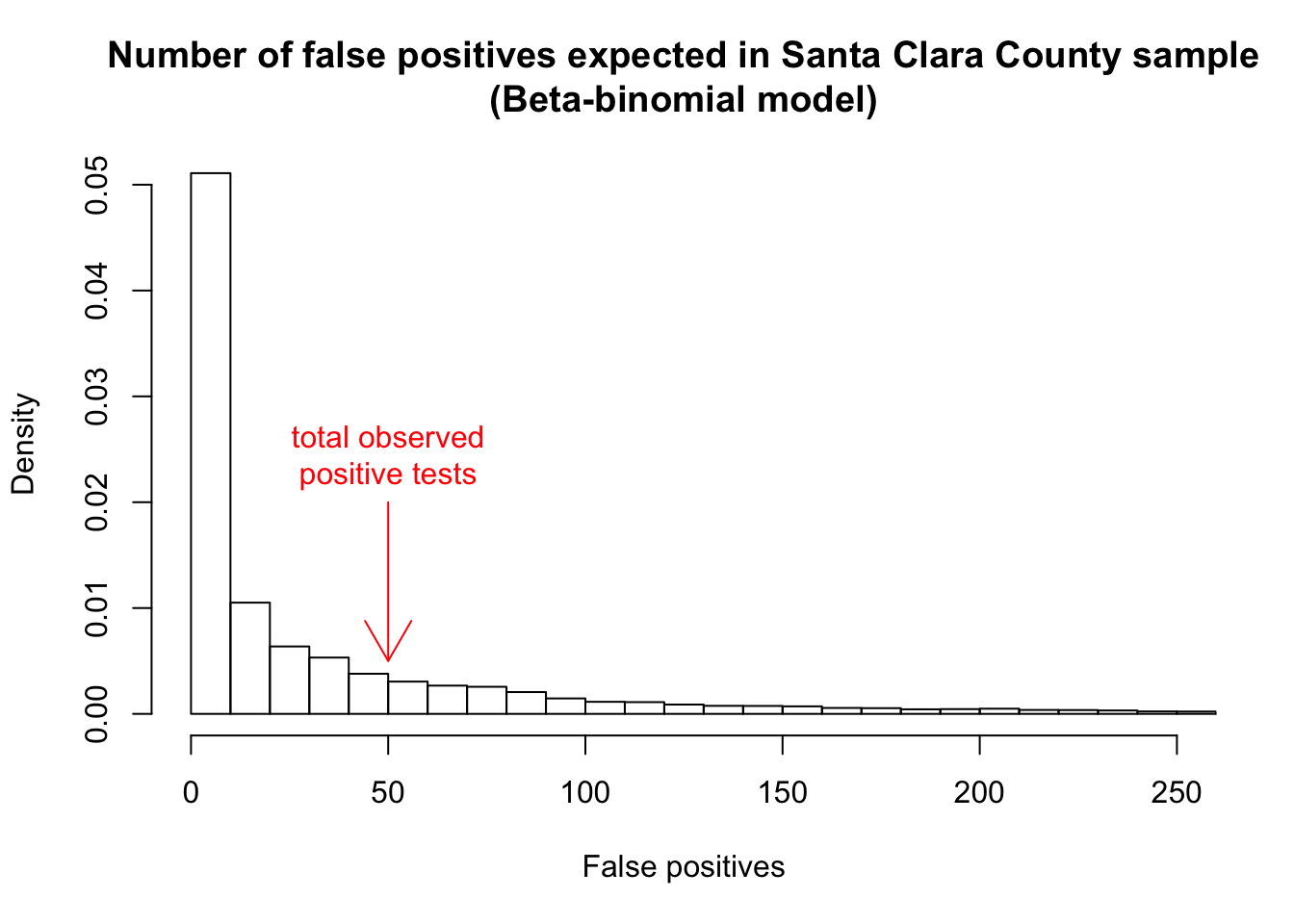This is a comment based on my Twitter thread, about why I still think the (unweighted) data analysis does not support the conclusion that the prevalence of COVID-19 in Santa Clara County is above zero. Of course, we know based on information from outside of the study that the prevalence is higher than zero – the point is that if the data in the study cannot even establish that, then they also cannot establish that the prevalence is large.

You can download the Rmd file here

## New data on specificity

The main new feature in the new study is the addition of 11 more negative control samples in addition to the two from the first draft, bringing the number of samples to 13 and providing more insight into the test’s specificity: $s = P(\text{test negative} \mid \text{true negative}).$

I find it a bit more intuitive to discuss things in terms of the test’s false positive rate (FPR), which is $$1-s$$. $\text{FPR} = P(\text{test positive} \mid \text{true negative}) = 1 - s.$ This is a population quantity, not a sample quantity: the percent of positive tests in any particular negative control sample is a noisy estimate of that sample’s true FPR.

Taken together, the 13 negative control samples have 3324 total specimens. We can copy the data into R, adding in the Santa Clara County study with 50 positives out of 3330 tests:

dat <- data.frame(
size     = c(371, 30, 70, 1102, 300, 311, 500, 200, 99, 31, 150, 108, 52, 3330),
test.neg = c(368, 30, 70, 1102, 300, 311, 500, 198, 99, 29, 146, 105, 50, 3280)
)

dat$test.pos <- dat$size - dat$test.neg dat$pct.pos <- round(dat$test.pos / dat$size * 100, 2)
dat$sample.type <- as.factor(c(rep("control", 13), "scc")) dat ## size test.neg test.pos pct.pos sample.type ## 1 371 368 3 0.81 control ## 2 30 30 0 0.00 control ## 3 70 70 0 0.00 control ## 4 1102 1102 0 0.00 control ## 5 300 300 0 0.00 control ## 6 311 311 0 0.00 control ## 7 500 500 0 0.00 control ## 8 200 198 2 1.00 control ## 9 99 99 0 0.00 control ## 10 31 29 2 6.45 control ## 11 150 146 4 2.67 control ## 12 108 105 3 2.78 control ## 13 52 50 2 3.85 control ## 14 3330 3280 50 1.50 scc Right away we see something odd: four of the 13 negative control samples have a higher fraction of false positives than the Santa Clara County sample. Looking at the data this way, it would be easy to believe that the Santa Clara County dataset was just one more negative control sample with a false positive rate somewhere in the middle of the pack. ## A heroic assumption? But Bendavid et al. don’t look at the data this way: they just pool the 13 negative control samples into one giant sample with 0.5% false positives overall. This makes sense only if we are assuming that all 14 of the samples have the same false positive rate.1 But that assumption doesn’t fit the data. In particular, we can reject it using Pearson’s $$\chi^2$$ test on the 13 x 2 table of negative control samples: (ctrl.tbl <- as.matrix(dat[dat$sample.type == "control", c("test.pos", "test.neg")]))
##    test.pos test.neg
## 1         3      368
## 2         0       30
## 3         0       70
## 4         0     1102
## 5         0      300
## 6         0      311
## 7         0      500
## 8         2      198
## 9         0       99
## 10        2       29
## 11        4      146
## 12        3      105
## 13        2       50
chisq.test(ctrl.tbl)
## Warning in chisq.test(ctrl.tbl): Chi-squared approximation may be incorrect
##
##  Pearson's Chi-squared test
##
## data:  ctrl.tbl
## X-squared = 75.819, df = 12, p-value = 2.571e-11

Because of the small counts we really should do a permutation test. This is computationally intensive.

perm.groups <- as.factor(rep(1:13, dat$size[1:13])) perm.labels <- rep(c(TRUE,FALSE), c(sum(dat$test.pos[1:13]),sum(dat$test.neg[1:13]))) perm.dist <- replicate(1E4, { perm.pos <- table(perm.groups[sample(perm.labels)]) perm.tbl <- cbind(perm.pos, dat$size[1:13] - perm.pos)
suppressWarnings(chisq.test(perm.tbl)$statistic) }) observed.stat <- suppressWarnings(chisq.test(ctrl.tbl)$statistic)

We can plot the permutation distribution:

hist(perm.dist, breaks=100, xlim=c(0, max(perm.dist, observed.stat)), freq=FALSE,
main = "Permutation Chi Squared Distribution",
xlab="Chi Squared Statistic")
arrows(observed.stat, .02, observed.stat, 0, col="red")
text(observed.stat, .02, "observed", pos = 3, col = "red")The permutation $$p$$-value:

1 - (rank(c(observed.stat, perm.dist)) - 1) / (1+length(perm.dist))
##  X-squared
## 0.00019998

## Modeling with overdispersed counts

The 13 samples are overdispersed relative to the binomial. One simple analysis is to fit a beta-binomial model to the negative control samples, using the VGAM library:

library(VGAM)
## Warning: package 'VGAM' was built under R version 3.6.2
## Loading required package: stats4
## Loading required package: splines
ctrl.bbfit <- vglm(ctrl.tbl ~ 1, family=betabinomial)

# back out
mu <- 1/(1+exp(-coef(ctrl.bbfit)))
rho <- 1/(1+exp(-coef(ctrl.bbfit)))
a.plus.b <- 1/rho - 1
a <- a.plus.b * mu
b <- a.plus.b * (1-mu)

# simulate FPRs
sim.fpr <- rbeta(10000, shape1 = a, shape2 = b)
sim.count <- rbinom(10000, sim.fpr, size = 3330)
hist(sim.count, breaks=100, xlim=c(0,250), freq = FALSE,
main = "Number of false positives expected in Santa Clara County sample\n(Beta-binomial model)", xlab="False positives")
arrows(50, .02, 50, 0.005, col="red")
text(50, .02, "total observed\npositive tests", pos = 3, col = "red")50 is the 77th percentile of this distribution, so there is again no way to be confident that any of the positive test kits represented true positives.

A bit more formally, there are a few simple ways to estimate binomial regression models with overdispersed counts. We can add an indicator variable of the sample type being Santa Clara County instead of control, and see if the coefficient is significant.

full.tbl <- as.matrix(dat[, c("test.pos", "test.neg")])

full.bbfit <- vglm(full.tbl ~ sample.type, data = dat, family=betabinomial)
summary(full.bbfit)
##
## Call:
## vglm(formula = full.tbl ~ sample.type, family = betabinomial,
##     data = dat)
##
## Pearson residuals:
##                   Min      1Q  Median     3Q    Max
## logitlink(mu)  -1.165 -0.8636 -0.3243 1.0652 2.3340
## logitlink(rho) -1.132 -0.6842  0.1456 0.5264 0.9328
##
## Coefficients:
##                Estimate Std. Error z value Pr(>|z|)
## (Intercept):1   -4.6025     0.5019  -9.170  < 2e-16 ***
## (Intercept):2   -3.6014     0.7212  -4.993 5.94e-07 ***
## sample.typescc   0.9995     0.9112   1.097    0.273
## ---
## Signif. codes:  0 '***' 0.001 '**' 0.01 '*' 0.05 '.' 0.1 ' ' 1
##
## Names of linear predictors: logitlink(mu), logitlink(rho)
##
## Log-likelihood: -27.4014 on 25 degrees of freedom
##
## Number of Fisher scoring iterations: 7
##
## Warning: Hauck-Donner effect detected in the following estimate(s):
## '(Intercept):1'
full.qbfit <- glm(full.tbl ~ sample.type, data = dat, family=quasibinomial)
summary(full.qbfit)
##
## Call:
## glm(formula = full.tbl ~ sample.type, family = quasibinomial,
##     data = dat)
##
## Deviance Residuals:
##    Min      1Q  Median      3Q     Max
## -3.261  -1.520  -0.269   1.887   2.686
##
## Coefficients:
##                Estimate Std. Error t value Pr(>|t|)
## (Intercept)     -5.3315     0.6299  -8.464  2.1e-06 ***
## sample.typescc   1.1479     0.7246   1.584    0.139
## ---
## Signif. codes:  0 '***' 0.001 '**' 0.01 '*' 0.05 '.' 0.1 ' ' 1
##
## (Dispersion parameter for quasibinomial family taken to be 6.318299)
##
##     Null deviance: 67.813  on 13  degrees of freedom
## Residual deviance: 49.314  on 12  degrees of freedom
## AIC: NA
##
## Number of Fisher Scoring iterations: 6
library(lme4)
## Loading required package: Matrix
dat$id <- as.factor(1:14) full.lmefit <- glmer(full.tbl ~ sample.type + (1 | id), data = dat, family = binomial) summary(full.lmefit) ## Generalized linear mixed model fit by maximum likelihood (Laplace ## Approximation) [glmerMod] ## Family: binomial ( logit ) ## Formula: full.tbl ~ sample.type + (1 | id) ## Data: dat ## ## AIC BIC logLik deviance df.resid ## 64.0 65.9 -29.0 58.0 11 ## ## Scaled residuals: ## Min 1Q Median 3Q Max ## -0.7753 -0.5524 -0.1408 0.3205 0.7618 ## ## Random effects: ## Groups Name Variance Std.Dev. ## id (Intercept) 3.033 1.742 ## Number of obs: 14, groups: id, 14 ## ## Fixed effects: ## Estimate Std. Error z value Pr(>|z|) ## (Intercept) -5.6919 0.7219 -7.885 3.16e-15 *** ## sample.typescc 1.4986 1.8907 0.793 0.428 ## --- ## Signif. codes: 0 '***' 0.001 '**' 0.01 '*' 0.05 '.' 0.1 ' ' 1 ## ## Correlation of Fixed Effects: ## (Intr) ## smpl.typscc -0.382 Note that all of this analysis still underestimates the uncertainty because it doesn’t take into account the fact that some of the participants in the SCC study were parent-child pairs. Finally, this is not an endorsement of the rest of the statistics in the paper. I am not addressing the weighted analysis at all. I would be surprised if the weighted analysis could overturn this conclusion, but without seeing the data I’m reluctant to guess. ## Pre-COVID samples Some of the negative control samples were based on pre-COVID blood and others were based on clinically excluded patients during the pandemic. For good measure, I redo the permutation test for the Pre-COVID negative control samples only. Nothing much changes. precovid <- (1:13)[-c(6, 11, 13)] precovid.perm.groups <- as.factor(rep(precovid, dat$size[precovid]))
precovid.perm.labels <- rep(c(TRUE,FALSE), c(sum(dat$test.pos[precovid]),sum(dat$test.neg[precovid])))

precovid.perm.dist <- replicate(1E4, {
precovid.perm.pos <- table(precovid.perm.groups[sample(precovid.perm.labels)])
precovid.perm.tbl <- cbind(precovid.perm.pos, dat$size[precovid] - precovid.perm.pos) suppressWarnings(chisq.test(precovid.perm.tbl)$statistic)
})

precovid.observed.stat <- suppressWarnings(chisq.test(ctrl.tbl[precovid,])\$statistic)
hist(precovid.perm.dist, breaks=100, xlim=c(0, max(precovid.perm.dist, precovid.observed.stat)), freq=FALSE,
main = "Permutation Chi Squared Distribution (Pre-COVID samples only)",
xlab="Chi Squared Statistic")
arrows(precovid.observed.stat, .02, precovid.observed.stat, 0, col="red")
text(precovid.observed.stat, .02, "observed", pos = 3, col = "red")